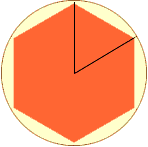Quandaries and Queries Who is asking: Teacher Level: Secondary Question: Is it possible for me to find any geometrical interpretation without using calculus to explain indeterminate forms? Thank you very much for your help. Hi, Here are two examples. Graph y = (x2 - 1) / (x-1) Inscribe a regular n-gon in a circle. The area of 1/n th of the circle (namely pi/n) is approximately equal to the area of 1/n th of the n-gon (namely (1/2)sin (2pi/n)). In other words, the ratio sin (2pi/n) over (2pi/n) goes to 1 as n goes to infinity.Chris Go to Math Central